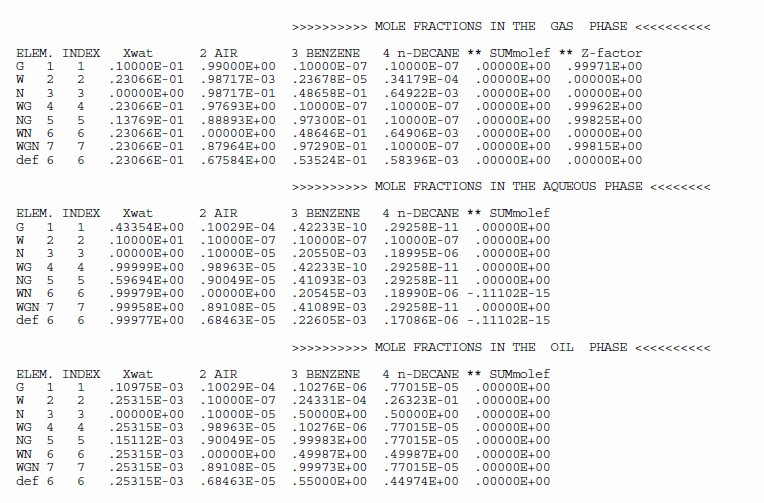0# Mole fraction in each phaseI am confused with term mole fraction in TMVOC output file.
I think the total of mole fractions in each phase in one element should equal to 1.

But, many output sample in TMVOC manual doesn't show that.
e.g., in problem 1 (*r7c*).

Does anyone can explain it?

Ito

2replies Oldest first
• Oldest first
• Popular•Hi Ito,

SUMMOLEF is not the sum of mole fractions, and is defined in the Users Guide in Section 9 "Output from TMVOC" as {sum of component mole fractions in a phase} -1. In this way, very small numbers of the difference (essentially numerical errors) can be represented. Yes, the variable name can be confusing.

The mole fractions add up to 1 if the phase is present for that grid block (e.g., the sum of mole fractions in the gas phase in G 1, that in the aqueous phase in W 2, and that in the oil phase in N 3). When a phase does not exist, the mole fractions of NCG and NHC are calculated based on the mole fractions of the existing phase using Henry's law. And the mole fraction of water is calculated by comparing pressure and saturated vapor pressure, for instance, between gas and aqueous phases. So the sum of the mole fractions of the non-existing phase does not necessarily add up to 1.

Hope this helps!

Yoojin
Like
•• ito
• ito
• 7 yrs ago
• Reported - view

Thank you, Yoojin.